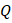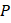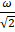# Two discsandare mounted coaxially on a vertical axle. The discs have moments of inertiaandrespectively about the common axis. Discis imparted an initial angular velocityusing the entire potential energy of a spring compressed by a distance. Discis imparted an angular velocityby a spring having the same spring constant and compressed by a distance. Both the discs rotate in the clockwise direction The loss of kinetic energy during the above process is a)b)c)d)## Question ID - 101407 :- Two discsandare mounted coaxially on a vertical axle. The discs have moments of inertiaandrespectively about the common axis. Discis imparted an initial angular velocityusing the entire potential energy of a spring compressed by a distance. Discis imparted an angular velocityby a spring having the same spring constant and compressed by a distance. Both the discs rotate in the clockwise direction The loss of kinetic energy during the above process is a)b)c)d)3537

(b)

Loss of kinetic energyNext Question :

The general motion of a rigid body can be considered to be a combination of (i) a motion---centre of mass about an axis, and (ii) its motion about an instantaneous axis passing through centre of mass. These axes need not be stationary. Consider, for example, a thin uniform welded (rigidly fixed) horizontally at its rim to a massless stick, as shown in the figure. Where disc-stick system is rotated about the origin on a horizontal frictionless plane with angular speed, the motion at any instant can be taken as a combination of (i) a rotation of the centre of mass the disc about the-axis, and (ii) a rotation of the disc through an instantaneous vertical axis pass through its centre of mass (as in seen from the changed orientation of pointsand). Both the motions have the same angular speedin the caseNow consider two similar systems as shown in the figure: casethe disc with its face vertical and parallel toplane; Casethe disc with its face making an angle ofwithplane its horizontal diameter parallel. In both the cases, the disc is welded at point, and systems are rotated with constant angular speedabout theWhich of the following statement regarding the angular speed about the instantaneous axis (passing through the centre of mass) is correct a) It isfor both the cases b) It isfor case; andfor casec) It isfor case; andfor cased) It isfor both the cases